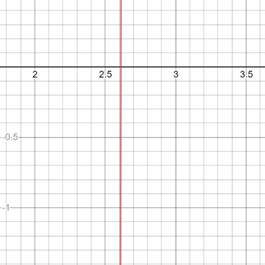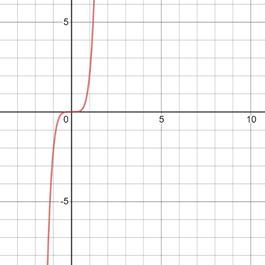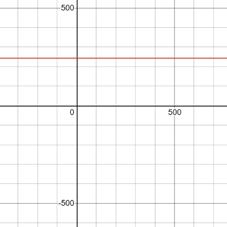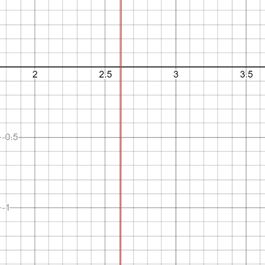# The value of x from the equation 2 x 5 − 243 = 0 by algebraic method and graphical method.### Precalculus: Mathematics for Calcu...

6th Edition
Stewart + 5 others
Publisher: Cengage Learning
ISBN: 9780840068071### Precalculus: Mathematics for Calcu...

6th Edition
Stewart + 5 others
Publisher: Cengage Learning
ISBN: 9780840068071

#### Solutions

Chapter 1.9, Problem 40E
To determine

## To calculate: The value of x from the equation 2x5−243=0 by algebraic method and graphical method.

Expert Solution

The value of x is 2.611 and the graph of the equation 2x5243=0 provided below,### Explanation of Solution

Given information:

The equation 2x5243=0 .

Calculation:

It is provided that the equation 2x5243=0 .

Algebraic method;

The method in which isolate x on one side of the equation and solve the value of x .

From the equation 2x5243=0 .

Add 265 on the both side of the equation,

2x5243+243=2432x5=243

Further solve for the value of x ,

x5=2432x=243215x=2.611

Therefore, the value of x=2.611 .

Graphical method;

Let put y1=2x5 and y2=243

The graph of the equation y1=2x5 provided below,The graph of equation y2=243 is provided below,The graph of both equations in same figure is provided below,The solution is the x coordinate of the intersection point of the two graphs. The intersection point on the x coordinate is 2.611 .

Thus, the value of x is 2.611 .

### Have a homework question?

Subscribe to bartleby learn! Ask subject matter experts 30 homework questions each month. Plus, you’ll have access to millions of step-by-step textbook answers!